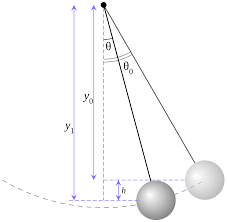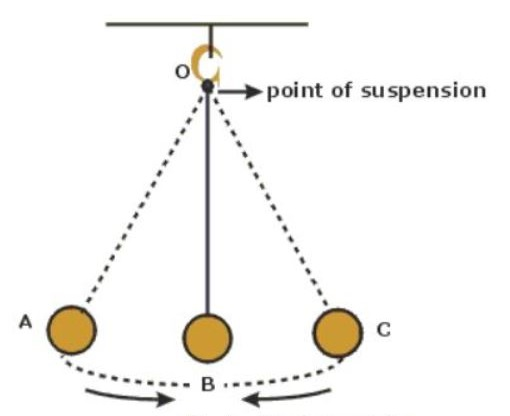# CBSE Class 11 Physics Chapter 14 Revision Notes

Chapter 14: Oscillations Revision Notes

• Periodic motions, processes, or phenomena are those that repeat themselves at regular intervals.

Oscillatory Motion:

• If a body moves to and fro around a fixed point at regular intervals of time, it is said to be oscillatory motion.
• The mean position or equilibrium position is the fixed point around which the body oscillates.

Simple Harmonic Motion is a type of periodic oscillatory motion in which the particle:

(i) oscillates along a straight line.

(ii) The particle’s acceleration is always directed at a fixed point on the line.

(iii) The particle’s displacement from the oscillations determines the magnitude of acceleration.Source

SHM Characteristics

x = A sin (ωt+ Ф) gives the displacement x in SHM at time t, where the three constants A, and distinguish one SHM from another. A cosine function can also be used to describe a SHM:

x = A cos (ωt + δ)

• At any point in time, the displacement of an oscillating particle equals the change in its position vector. “Displacement amplitude” or “simple amplitude” refers to the maximum value of displacement in an oscillatory motion on either side of its mean position.

As a result, amplitude A = x max.

• The “time period” of SHM refers to how long it takes an oscillating particle to complete one full oscillation to and fro around its mean (equilibrium) position.

• Frequency is defined as the number of oscillations per second. It is measured in seconds per second or Hertz per second. Amplitude has no bearing on frequency or time period.

• The quantity (ωt+ Ф) is known as the SHM phase at time t, and it describes the state of motion at that time. The quantity is the phase at time f = 0 and is referred to as the SHM’s phase constant, initial phase, or epoch. In the cosine or sine function, the phase constant is the time-independent term.

• The energy of a body that performs SHM alternates between kinetic and potential, but the total energy remains constant. When x is moved away from the equilibrium position:

• The spring constant of a combination is given by oscillations when two springs with spring constants k1 and k2 are connected in series.

• Parallel Springs: When one spring is attached to two masses m1 and m2, the spring constant of the combination is given by k = k1 + k2 • When one spring is attached to two masses m1 and m2, the spring constant of the combination is given by k = k1 + k2Source

• Pendulum with a Simple Design: The most common example of bodies executing S.H.M. is a simple pendulum. An ideal simple pendulum consists of a heavy point mass body suspended from a rigid support by a weightless, extensible, and perfectly flexible string. It is free to oscillate.

• A simple pendulum’s time period is determined by (i) the length of the pendulum and (ii) the acceleration due to gravity (g).

• A second’s pendulum has a time period of two seconds. The length of a second’s pendulum is found to be 99.3 cm (= 1 m) when g = 9.8 ms-2.

• If a liquid with density p oscillates in a vertical U-tube with uniform cross sectional area A, then oscillation is the time period of the oscillation.

• If a cylinder with mass m, length L, material density p, and uniform area of cross section A oscillates vertically in a liquid with density o, the time period of oscillation is given by oscillations with Simple Harmonics

• Undamped Simple Harmonic Oscillations: Undamped simple harmonic oscillations occur when a simple harmonic system oscillates with a constant amplitude that does not change with time.

• Damped Simple Harmonic Oscillations: Damped simple harmonic oscillations occur when a simple harmonic system oscillates with a decreasing amplitude over time.

• Forced oscillations occur when a system is compelled to oscillate at a frequency other than its natural frequency.

]]>